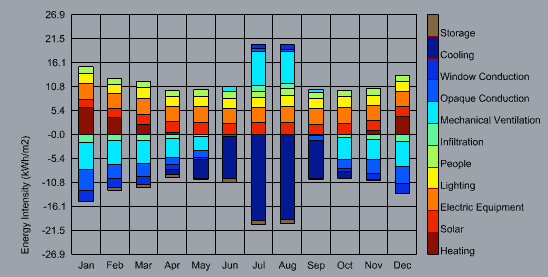Hello Everyone,

During a balance calculation using the EP component and the monthly chart, I get:Now, while using the new HB Peak Loads component, focusing on Heating Balance data, I can extract:
Infiltration, Window conduction and Opaque conduction. But I can’t find the Mechanical Ventilation data. Is it a bug or an intentional?

Additionally, for the Heating results. Would be possible to get the breakdown in Solar, People, Lighting, Heating,…

I understand all this can be extracted directly from the EP component. Just wondering if the Peak Load component could manage this additional information.

Thanks.

@Barbarur

Mechanical ventilation isn’t technically a zone load. In EnergyPlus, only ventilation loads that directly enter the zone from the outside is considered a zone load (i.e. through a window, or zone intake/exhaust fans), whereas the outdoor air from mechanical ventilation is passed through the HVAC air loop. Therefore the energy required to condition the outdoor air at the time of total peak load is incorporated into the required HVAC capacity calculations separately by EnergyPlus, it’s not part of any specific zone peak load calculation.

As for peak heating, the point of the “peak” calculation is to model an extreme heating scenario. As such the heat gain impact of solar, people, lighting, and equipment is discounted.

Thank you for the answer. I get a bit confuse with a couple points:

1. if EP can provide a balance chart including the machanical ventilation. How later we cannot get this value separately?

2. The Peak heating is calculated considering there will be solar, people, light and equipment providing heat. But the value only reflects the additional heating needed. is it right?

@Barbarur

Think of it this way: the cooling load in your “Peak Load” graph from components like solar, people, or lighting are a result of the heat gain directly inside your zone, i.e solar radiation is absorbed by zone material and released by convection into the zone air. The mechanical ventilation on the other hand is conditioned outside your zone, in the HVAC system. By the time the outdoor air is blown into your zone it is therefore not contributing to the zone peak load. Therefore the mechanical ventilation is not considered part of the zone peak load, it’s considered a system load.

However, each zone has its own outdoor air requirements that result in a specific cooling load associated with ventilation. Therefore when doing system sizing we still need to calculate it’s value and add it to the coincident zone peak load to determine the HVAC system capacity, and it still appears in the “Thermal Energy Balance Chart” since it is a component of the zone energy balance.

My own mental model of this is based on the zone air energy balance which EP uses to calculate system air flowrates. Basically, the energy supplied to the zone is equal to the energy difference between the system supply air T_{sa} and exiting zone air (or return air) T_{ra} (broken down below as the sum of the energy contributed by various zone components to the zone air):

\begin{align*} \dot{Q}_{zone} &= \sum_{i}^{N_{int}} \dot{Q}_i + \sum_{i}^{N_{srf}} h_i A_i \Delta{T} + \sum_{i}^{N_{zones}} \rho \dot{V}_i C \Delta{T} + \rho \dot{V}_{inf} C \Delta{T} \\ \\ &= \dot{V}_{ra} \rho C (T_{sa} - T_{ra}) \\ \end{align*}

You’ll notice that the energy required to condition the mechanical ventilation is not included at all. That’s a separate component, equal to the energy difference between the system supply air and the outdoor air.

\dot{Q}_{oa} = \dot{V}_{oa} \rho C_p (T_{sa} - T_{oa})

And the total system energy per zone is therefore equal to the sum of both:

\dot{Q}_{sys} = \dot{V}_{oa} \rho C_p (T_{sa} - T_{oa}) + \dot{V}_{ra} \rho C_p (T_{sa} - T_{ra})

All variables used above are defined in the EP Engineering Reference here: Basis for the Zone and Air System Integration: Engineering Reference — EnergyPlus 9.5.

Re: Peak heating. The peak heating is calculated without considering solar, people, light and equipment. All of those components are set to zero.